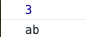# TypeScript（6）函数

• TypeScript（6）函数已关闭评论
• 13 次浏览
• A+

# 函数

## 基本示例

``// 命名函数 function add(x, y) {   return x + y }  // 匿名函数 let myAdd = function(x, y) {    return x + y; } ``

## 函数类型

### 为函数定义类型

``function add(x: number, y: number): number {   return x + y }  let myAdd = function(x: number, y: number): number {    return x + y } ``

### 书写完整函数类型

``// 函数的完整写法 // myAdd2---->变量名----函数myAdd2 //  (x: number, y: number) => number 是当前这个函数的类型 // function(x: number, y: number): number { return x + y } 相当于符合上面这个函数的值 let myAdd2: (x: number, y: number) => number =  function(x: number, y: number): number {   return x + y } ``

## 可选参数和默认参数

`TypeScript` 里的每个函数参数都是必须的。 这不是指不能传递 `null``undefined` 作为参数，而是说编译器检查用户是否为每个参数都传入了值。编译器还会假设只有这些参数会被传递进函数。 简短地说，传递给一个函数的参数个数必须与函数期望的参数个数一致。

`JavaScript` 里，每个参数都是可选的，可传可不传。 没传参的时候，它的值就是 `undefined`。 在TypeScript 里我们可以在参数名旁使用 `? `实现可选参数的功能。 比如，我们想让 `lastName` 是可选的

`TypeScript` 里，我们也可以为参数提供一个默认值当用户没有传递这个参数或传递的值是 `undefined` 时。 它们叫做有默认初始化值的参数。 让我们修改上例，把`firstName` 的默认值设置为` "A"`

``function buildName(firstName: string='A', lastName?: string): string {   if (lastName) {     return firstName + '-' + lastName   } else {     return firstName   } }  // 都传入 console.log(buildName('C', 'D')) // 只传入姓 console.log(buildName('C')) // 什么也不传 console.log(buildName()) ``## 剩余参数

``function info(x: string, ...args: string[]) {   console.log(x, args) } info('abc', 'c', 'b', 'a') ``## 函数重载

``/*  函数重载: 函数名相同, 而形参不同的多个函数 需求: 我们有一个add函数，它可以接收2个string类型的参数进行拼接，也可以接收2个number类型的参数进行相加  */  // 重载函数声明 function add (x: string, y: string): string function add (x: number, y: number): number  // 定义函数实现 function add(x: string | number, y: string | number): string | number {     // 在实现上我们要注意严格判断两个参数的类型是否相等，而不能简单的写一个 x + y     if (typeof x === 'string' && typeof y === 'string') {         return x + y     } else if (typeof x === 'number' && typeof y === 'number') {         return x + y     } }  console.log(add(1, 2)) console.log(add('a', 'b')) ``